# 洁净室一次回风处理过程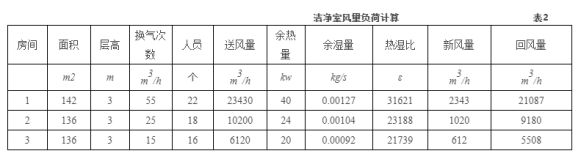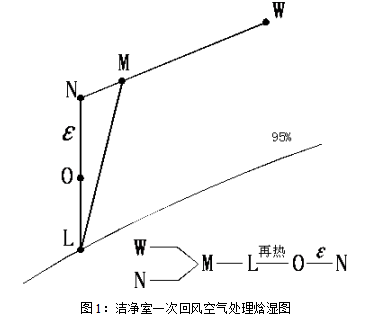Δh=Q/G

Δh：室内状态点同送风状态点的焓差（in-io）；

Q：室内余热；

G：房间送风量；

Δh=40/7.81

=5.12kJ/kg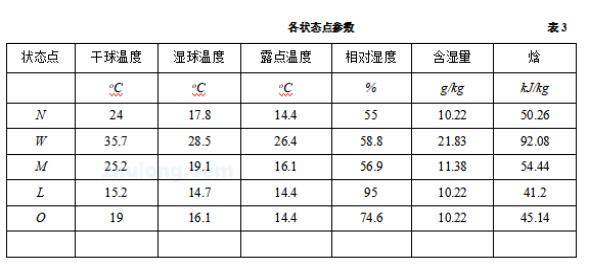=7.81*5.12

=40kw

=0.781*41.82

=32.7kw

=7.81*3.94

=30.77kw

=40+32.7+30.77

= 103.5kw

G=Q/in-il

=30/(50.26-41.2)

=3.31kg/s9933m3/h

=13.84kw

2DDC自动控制原理图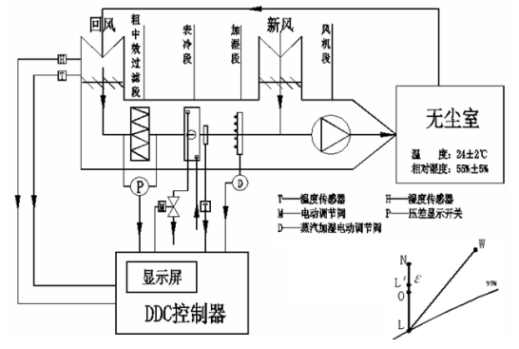安装在回风管内的温度传感器T检测的温度送至DDC与设定的点相比较，用比例积分控制，输出相应的电压控制电动调节阀M的开度，从而精确调节冻冻水流量，使送风温度保持在所需要的范围内。

3：方案1空气处理焓湿图                  4：方案2空气处理焓湿图

=0.781*50.88

=39.7kw

=7.03*4.68

=32.9kw

=39.7+32.9

=72.9kw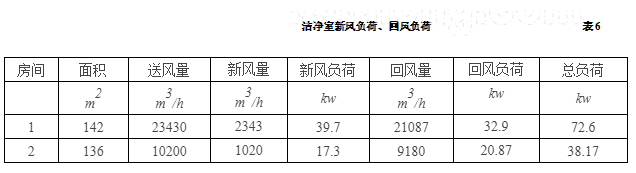通过观察可知：总冷负荷与再热式一次回风系统的室内负荷+新风负荷的总和相等，即用些种空气处理方式省掉了再热量,同时还有相应等量的冷量,节能效益相当可观.

DDC控制原理图：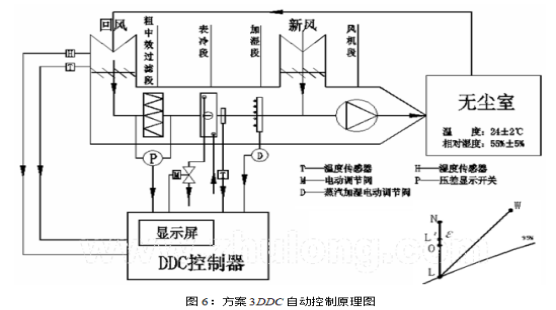GB 50073-2001 洁净厂房设计规范.

北京一林嘉业